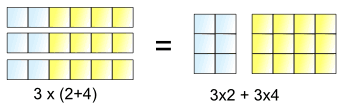Definition of

# Distributive Law

The Distributive Law says that multiplying a number by a group of numbers added together is the same as doing each multiplication separately.

Example: 3 × (2 + 4) = 3×2 + 3×4

So the "3" can be "distributed" across the "2+4" into 3 times 2 and 3 times 4.